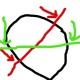# [closed] What solver for LP with single non-linear constraint?

 2 Hi all, I'm trying to solve the problem exposed at the end of section 2 of this paper. For simplicity I reproduce it here: $\begin{matrix} \min & \sum_{i} u_i & + & v_i\\ \text{s.t.} & \sum_j a_j x_{ij} & + & u_i - v_i & \leq & c, & \quad i=1,\ldots, n\\ & & & v_i, ~u_i & \geq & 0, & \quad i=1,\ldots, n\\ & & & \prod_j a_j & = & \pm{1} \end{matrix}$ the last constraint is the one i have a problem with (note that the $$a_j$$s are not constrained to be positive). The author claims this problem is convex (because of the fact that the $$a_j$$s are not constrained positive this is not obvious to me). At any rate, would you know of an academic friendly solver (either open-source or CPLEX) that could solve this type of problems? Or maybe this problem can be rewritten in standard form? Thanks in advance asked 04 Jan '12, 08:38vak 33●1●4 accept rate: 0%fbahr ♦ 4.6k●7●16

### The question has been closed for the following reason "The question is answered, right answer was accepted" by fbahr 16 Jan '14, 07:14

 5 Are the $$x_{ij}$$ parameters? If not, the inequality constraints are quadratic (and nonconvex). If the $$a_j$$ are unrestricted in sign, then your feasible region is clearly nonconvex because it omits any point with any $$a_j = 0$$ but includes neighborhoods around those points. The formulation looks "off" to me in any case. Let's assume the $$x_{ij}$$ are sample data and the $$a_j$$ are regression coefficients (to be determined). Now suppose that we change the signs of all observed values. I would expect that changing the signs of all the coefficients of the previous optimal fit would be the new optimal fit; but if the number of regression variables (dimension of $$a$$) is odd, this won't work, because the product will be -1 rather than 1. answered 04 Jan '12, 13:42Paul Rubin ♦♦ 14.6k●5●13 accept rate: 19%fbahr ♦ 4.6k●7●16 The paper at hand is on curve fitting in m-dimensional space. (It's by Chris Tofallis, thus: I only understand half of it - since he uses 's' as if it was a 'z'.) The $$x_{i,j}$$s are data points ($$i=1,..,n; j=1,..,m$$), so the only non-linear constraint is last one, $$\prod \limits_{j} a_j = \mathbf{\pm} 1$$. (04 Jan '12, 14:42) fbahr ♦ 1 If they specify $$\prod_j a_j=\pm 1$$ then I'm less skeptical about the formulation (but the feasible region is still nonconvex). (04 Jan '12, 14:52) Paul Rubin ♦♦ No clue whether it's true or not, but here's what the paper actually states: "For fitted functions that are linear in the coefficients[,] the optimisation method for their estimation can be shown to be a convex fractional programme so that any optimum is both unique and global." [No proof or reference given.] And: "Whilst there are solution algorithms for fractional programmes, the unique global property implies that general convex solution methods can be used." (04 Jan '12, 15:02) fbahr ♦ I think the author might have a wrong understanding of fractional programming as it requires denominator to be positive, but $$a_{j}$$s are URS. (04 Jan '12, 15:09) Ehsan ♦ @Paul Rubin: Sorry for not posting the full model (with the double +/- constraints): i tried at first but the latex support would not work and it was becoming too confusing. Thanks for confirming that the feasible set is not convex. (05 Jan '12, 04:59) vak
 4 Your nonlinear constraint is not quadratic, hence, you could not solve your problem using CPLEX or Gurobi. I recommend using LINGO because of its powerful nonlinear solver and easy interface. Regarding the academic licence option, I think its developer gives 6-month temporary educational research license key with no size limit. I've got one from them recently, however, I could not find any link on their website about this right now. You could ask their sales office if these licence keys are still offered. answered 04 Jan '12, 11:53Ehsan ♦ 4.8k●3●11●24 accept rate: 16%
 2 You may also try Couenne. It is open-source, and works well. It is also available at NEOS (http://www.neos-server.org/neos/solvers/minco:Couenne/AMPL.html), so you don't even have to install anything. Just submit the problem in AMPL or GAMS format. If you are solving the problem numerically, and if you assume ( a_i geq 0 ) you may want to rewrite the nonlinear constraint as ( sum_i(log a_i) = 0 ). It may work better for the solver. answered 08 Jan '12, 20:22ashutosh mah... 150●6 accept rate: 0% thanks for the link (unfortunatly, i can't assume a_i>0....) (15 Jan '12, 13:47) vak

By Email:

Markdown Basics

• *italic* or _italic_
• **bold** or __bold__
• image?![alt text](/path/img.jpg "Title")
• numbered list: 1. Foo 2. Bar
• to add a line break simply add two spaces to where you would like the new line to be.
• basic HTML tags are also supported

Tags: